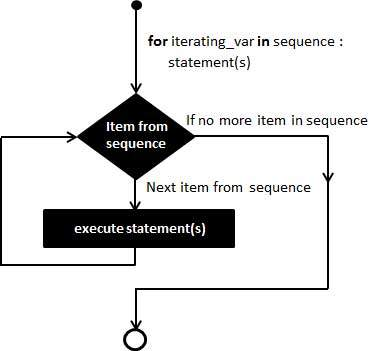# Python for Loop Statements

It has the ability to iterate over the items of any sequence, such as a list or a string.

### Syntax

```for iterating_var in sequence:
statements(s)
```

If a sequence contains an expression list, it is evaluated first. Then, the first item in the sequence is assigned to the iterating variable iterating_var. Next, the statements block is executed. Each item in the list is assigned to iterating_var, and the statement(s) block is executed until the entire sequence is exhausted.

### Flow Diagram### Example

Live Demo
```#!/usr/bin/python

for letter in 'Python':     # First Example
print 'Current Letter :', letter

fruits = ['banana', 'apple',  'mango']
for fruit in fruits:        # Second Example
print 'Current fruit :', fruit

print "Good bye!"
```

When the above code is executed, it produces the following result −

```Current Letter : P
Current Letter : y
Current Letter : t
Current Letter : h
Current Letter : o
Current Letter : n
Current fruit : banana
Current fruit : apple
Current fruit : mango
Good bye!
```

## Iterating by Sequence Index

An alternative way of iterating through each item is by index offset into the sequence itself. Following is a simple example −

Live Demo
```#!/usr/bin/python

fruits = ['banana', 'apple',  'mango']
for index in range(len(fruits)):
print 'Current fruit :', fruits[index]

print "Good bye!"
```

When the above code is executed, it produces the following result −

```Current fruit : banana
Current fruit : apple
Current fruit : mango
Good bye!
```

Here, we took the assistance of the len() built-in function, which provides the total number of elements in the tuple as well as the range() built-in function to give us the actual sequence to iterate over.

## Using else Statement with For Loop

Python supports to have an else statement associated with a loop statement

• If the else statement is used with a for loop, the else statement is executed when the loop has exhausted iterating the list.

The following example illustrates the combination of an else statement with a for statement that searches for prime numbers from 10 through 20.

Live Demo
```#!/usr/bin/python

for num in range(10,20):     #to iterate between 10 to 20
for i in range(2,num):    #to iterate on the factors of the number
if num%i == 0:         #to determine the first factor
j=num/i             #to calculate the second factor
print '%d equals %d * %d' % (num,i,j)
break #to move to the next number, the #first FOR
else:                  # else part of the loop
print num, 'is a prime number'
break
```

When the above code is executed, it produces the following result −

```10 equals 2 * 5
11 is a prime number
12 equals 2 * 6
13 is a prime number
14 equals 2 * 7
15 equals 3 * 5
16 equals 2 * 8
17 is a prime number
18 equals 2 * 9
19 is a prime number
```
python_loops.htm